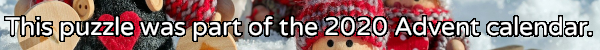mscroggs.co.uk
mscroggs.co.uksubscribe

# Puzzles

## 7 DecemberThere are 15 dominos that can be made using the numbers 0 to 4 (inclusive):
The sum of all the numbers on all these dominos is 60.
Today's number is the sum of all the numbers on all the dominos that can be made using the numbers 5 to 10 (inclusive).

## 4 DecemberThere are 5 ways to tile a 3×2 rectangle with 2×2 squares and 2×1 dominos.
Today's number is the number of ways to tile a 9×2 rectangle with 2×2 squares and 2×1 dominos.

## Archive

Show me a random puzzle
▼ show ▼